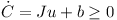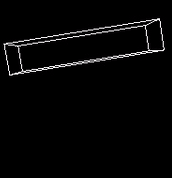# Rigid body game physics 4

The following article is partially based on Hubert Eichner’s article on inequality constraints.

## Inequality Constraints for Colliding Contacts

At a colliding contact the relative speed of the two objects is negative. I.e. a contact is a colliding (and not a resting) contact if the normal speed at the contact point is below a small negative threshold. Colliding contacts have restitution, i.e. the collision is partially elastic and the two objects will separate after the collision. The colliding contacts and affected joints are handled using a special time step of zero duration. Again the inequality constraint isThe linear and angular components of J are the same as for a resting contact. The correction term b however depends on the initial normal speed at the contact point vn and the restitution coefficient ε:## Sequential Impulses for Collisions

Again the contact and joint impulses are estimated iteratively. Note that the normal speed vn at each contact is determined beforehand and stays constant during the iterations.

• determine vn for all colliding contacts
• for each iteration
• for each joint
1. compute Jacobian J and correction vector b
2. predict speed u
3. compute λ
4. compute the impulse P
5. add P to accumulated impulses of the two objects
• for each colliding contact
1. subtract old impulses P from previous iteration from accumulated impulses of the two objects
2. compute Jacobian J and correction vector b
3. predict speed u
4. compute new λ and clip it to be greater or equal to zero
5. compute the impulse P
6. add P to accumulated impulses of the two objects
• apply impulses to objects

The value λ is stored as Pn for limiting friction impulses lateron. The time step here is zero! Therefore external forces do not need to be considered while handling collisions. An object falling to the floor will experience several collisions until the linear and angular speed has decreased sufficiently for the contacts to become resting contacts.